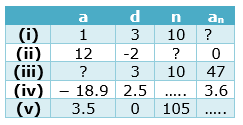# Class 10 Maths Assignments for Arithmetic Progression

Given below are the Class 10 Maths Worksheet for Arithmetic Progression
a. Fill in the blanks
b. Calculation problems
c. True & False Questions
d. Multiple Choice Questions

## Fill in the blanks

Question 1.
Fill in the blanks in the following table, given that a is the first term,d the common difference and thenthterm of the A.P.## Calculation Problems

Question 2.
Which of the following sequences are A. P’S?
Write down their common difference.
(a) 5, 10, 15, 20,25......
(b) 2, 2, 2, 2,2......
(c) 2, 4, 8, 16,32......
(d) 3, 14, 25, 36......
(e)11, 8, 5, 2, .....

Question 3
Write next two terms of given A.P. -3, -5, -7,-9......

Question 4
The sum of the 4th and 8th terms of an AP is 24 and the sum of the 6th and the 10th terms is 44. Find the first three terms of the AP

## True and False

Question 5
True and False statement
a. 10th term of A.P. 10, 20, 30, 40 ......is 100
b. 20th term of A.P. whose first term = 10, common difference = 3 is 67
c. The number of bacteria in a certain food item after each second forms an AP. when they double in every second
d. The amount of money in the account of Varun at the end of every year forms an AP when Rs 1000 is deposited at simple interest of 10% per annum
e. The fee charged every month by a school from Classes I to XII forms an AP, when the monthly fee for Class I is Rs 250, and it increases by Rs 50 for the next higher class.
f. The fee charged from a student every month by a school for the whole session forms an AP when the monthly fee is Rs 400.

## Multiple Choice Questions

Question 6
For what value of a, are (2a-1), 7 and 3a three consecutive terms of an A.P?
(a) 6
(b) 3
(c) 2
(d) 8

Question 7
How many multiples of 4 lie between 10 and 250?
(a) 66
(b) 65
(c) 60
(d) 64

Question 8
Find the value of p, so that (3p + 7), (2p+5), (2p + 7) are in A.P
(a) 5
(b) -5
(c) 4
(d) -4

Question 9
If Sn, the sum of first n terms of an A.P is given by
$S_n = 3n^2 - 4n$
then find its nth term
(a) 5n + 8
(b) 6n +1
(c) 6n -7
(d) 6n -8

Question 10
Find the sum of the following A.P 1 + 3 + 5 + ..... + 199.

(a) 10000
(b) 10500
(c) 11000
(d) 10800

Question 11
Solve the equation:
$1 + 4 + 7 + 10 +...+ x =287$
(a) 40
(b) 41
(c) 59
(d) 54

Question 12
If the common difference of an AP is 5, then what is $a_{18} -a_{13}$?
(a) 40
(b) 25
(c) 5
(d) 10

Question 13
If the numbers n � 2, 4n � 1 and 5n + 2 are in AP, find the value of n.
(a) -1
(b) 2
(c) 1
(d) 0

Question 14
Find the sum
$\frac {x- y}{x+y} + \frac {3x- 2y}{x+y} + \frac {5x- 3y}{x+y} +$..upto 11 terms
(a) $\frac {11(11x+ 6y)}{x+y}$
(b)$\frac {11(10x- 6y)}{x+y}$
(c) $\frac {11(12x- 6y)}{x+y}$
(d) $\frac {11(11x- 6y)}{x+y}$

## Calculation Problems

Question 15
Find the value of the middle most term (s) of the AP
-11, -7, -3,..., 49

Question 16
The 4th term of an A.P is equal to 3 times the first term and the 7th term exceeds twice the 3rd term by 1. Find the A.P

Question 17
The sum of n terms of an A.P. is $3n^2 + 5n$. Find the A.P. Hence, find its 16th term

Question 18
The angles of a triangle are in A.P, the least being half the greatest. Find the angles

Question 19
Find the
(i) sum of those integers between 1 and 500 which are multiples of 2 as well as of 5.
(ii) sum of those integers from 1 to 500 which are multiples of 2 or 5

Question 20
Find the sum of all three digit numbers which leave the remainder 3 when divided by 5

## Summary

This Class 10 Maths Worksheet for Arithmetic Progression with answers is prepared keeping in mind the latest syllabus of CBSE . This has been designed in a way to improve the academic performance of the students. If you find mistakes , please do provide the feedback on the mail.You can download in PDF form also using the below linksGo back to Class 10 Main Page using below links

### Practice Question

Question 1 What is $1 - \sqrt {3}$ ?
A) Non terminating repeating
B) Non terminating non repeating
C) Terminating
D) None of the above
Question 2 The volume of the largest right circular cone that can be cut out from a cube of edge 4.2 cm is?
A) 19.4 cm3
B) 12 cm3
C) 78.6 cm3
D) 58.2 cm3
Question 3 The sum of the first three terms of an AP is 33. If the product of the first and the third term exceeds the second term by 29, the AP is ?
A) 2 ,21,11
B) 1,10,19
C) -1 ,8,17
D) 2 ,11,20